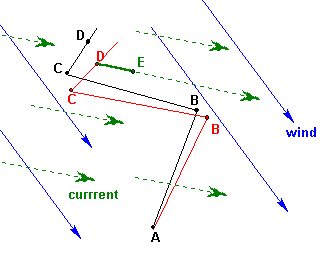|| home || coastal nav support || support home || sitemap ||

Correcting DR for leeway and current.

 The black line A,B,C,D is the DR plot without corrections To correct for leeway, use same distance run for each leg, but plot each course leg to leeward of the actual course steered by an amount equal to the estimated or measured leeway angle. This is the red line. Note length AB = AB, BC = BC, etc, it is just the course angles that are offset. Then correct for the current set at the end of the plot, by moving the final position by the amount set during the time of the run. In this case, the green line DE, is the drift which we move in the direction of the set. If T is the total time in decimal hours from A to D and Cur is the current speed in kts, then this total drift in nmi DE = T x Cur. Note: i will later fix the plot. Point A should be marked with a circle around a cross, B,C,D, with half circles, and E with a square.Assumptions: (1) The wind speed and direction are constant over the plot and we are on the same point of sail on each leg, and (2) the set and drift of the current are constant over the region of the plot.

|| home || coastal nav support || support home || sitemap ||